# A Non-Dimensional Analysis of Hemodialysis

Michael Bonert1, Bradley A Saville*, 2
1 Department of Laboratory Medicine and Pathobiology, University of Toronto, Canada
2 Department of Chemical Engineering, University of Toronto, Canada

#### Article Metrics

0
##### Total Statistics:

Full-Text HTML Views: 1353
Abstract HTML Views: 899
##### Unique Statistics:

Full-Text HTML Views: 666
Abstract HTML Views: 532

© Bonert and Saville; Licensee Bentham Open.

open-access license: This is an open access article licensed under the terms of the Creative Commons Attribution Non-Commercial License (http://creativecommons.org/licenses/by-nc/3.0/) which permits unrestricted, non-commercial use, distribution and reproduction in any medium, provided the work is properly cited.

* Address correspondence to this author at the Department of Chemical Engineering and Applied Chemistry, University of Toronto, 200 College Street, Toronto, Ontario, M5S 3E5, Canada; Tel: 416-978-7745; Fax: 416-978-8605; E-mail: bradley.saville@utoronto.ca

## Abstract

### Background:

Non-dimensional analysis is a powerful approach that can be applied to multivariate problems to better understand their behaviour and interpret complex interactions of variables. It is has not been rigorously applied to the parameters that define renal dialysis treatments and may provide insight into the planning of hemodialysis treatments.

### Methods:

Buckingham’s non-dimensional approach was applied to the parameters that define hemodialysis treatments. Non-dimensional groups were derived with knowledge of a mass transfer model and independent of it. Using a mass transfer model, the derived non-dimensional groups were plotted to develop an understanding of key relationships governing hemodialysis and toxin profiles in patients with end-stage renal disease.

### Results:

Three non-dimensional groups are sufficient to describe hemodialysis, if there is no residual renal function (RRF). The non-dimensional groups found represent (1) the number of half-lives that characterize the mass transfer, (2) the toxin concentration divided by the rise in toxin concentration without dialysis for the cycle time (the inverse of the dialysis frequency), and (3) the ratio of dialysis time to the cycle time. If there is RRF, one additional non-dimensional group is needed (the ratio between cycle time and intradialytic elimination rate constant). Alternate non-dimensional groups can be derived from the four unique groups.

### Conclusions:

Physical interpretation of the non-dimensional groups allows for greater insight into the parameters that determine dialysis effectiveness. This technique can be applied to any toxin and facilitates a greater understanding of dialysis treatment options. Quantitative measures of dialysis adequacy should be based on dimensional variables.

Keywords: Dialysis adequacy, non-dimensional analysis, dimensionless groups, hemodialysis, peritoneal dialysis, dialysis dose, Buckingham Pi theorem, Kt/V..

## INTRODUCTION

Since the beginning of clinical hemodialysis by Willem Kolff in the 1940s, much has been written about how to measure dialysis and how much dialysis ought to be given. Shinaberger has written a historical perspective on this, from the non-technical perspective .

Numerous mass transfer models [2-4] have been developed to understand hemodialysis and can reproduce hemodialysis quite well. However, it remains a difficult area to understand without resorting to calculations which, practically, require a computer. This creates challenges when (1) deciding how patients should be treated and (2) studying the pathophysiology of end-stage kidney failure.

Fortunately, a number of tools exist to analyze complex multi-variate problems and simplify them, as these are very common in engineering. One in particular, grouping variables into non-dimensional groups, has proven effective and has a long history.

Non-dimensional grouping simplifies the analysis of complex problems by splitting the problem into two stages:

1. grouping the dimensional variables (into non-dimensional groups) and
2. relating the non-dimensional groups.

While non-dimensional groups have a physical interpretation and are often significant on their own, they do not replace dimensional values.

In 1914, Buckingham derived a theorem [5, 6], now known as the Buckingham Pi Theorem, which formalized the procedure for deriving non-dimensional groups. The power of the theorem is that one does not need prior knowledge of how the physical quantities in a given problem are related; one only needs to make an educated guess as to which quantities are relevant to the problem. If the equation which describes a given problem is known, it can aid in the physical interpretation of the non-dimensional groups. Also, equation(s), if non-empirical, can be used to derive non-dimensional groups, with a small amount of algebra .

The resulting dimensionless groups are a convenient means for scaling of processes and experiments [8, 9].

In complex systems, as Buckingham demonstrated, several unique sets of non-dimensional groups may be formed and transformations between these groups may allow one to gain insights into the physical system and/or develop better experimental designs . If the relationships between the non-dimensional groups are known (by measurement or by theory) it may be possible to plot a multivariate problem with six or seven dimensional variables on one 2-dimensional plot in non-dimensional form, as done for pipe flow (which is a six variable problem) .

Although dimensionless groups have been used to quantify the effectiveness of dialysis treatments, the non-dimensional groups have not been formally derived, and their significance remains to be developed.

Furthermore, the relationship between key parameters and the non-dimensional groups has not been explored, and their relationship to concentration is not apparent in the literature. The goal of this work is to develop the relevant non-dimensional groups for renal dialysis, in an effort to improve our understanding of key parameters that lead to effective dialysis.

### Pseudo Non-Dimensional Groups in Hemodialysis

Kt/V, which appeared in Babb’s 1971 paper , was recognized as important when Gotch and Sargent re-analyzed the data from NCDS . However, Gotch and Sargent did not treat Kt/V as a non-dimensional group; they cast it as measure of dialysis and a substitute for urea concentration. At the time, this was an advance as urea concentration is a poor measure of dialysis because it is strongly dependent on protein intake.

Motivated by the solution of the exponential decay (differential) equation, Gotch defined Kt/V as: 

Equation 1: Definition of Gotch's Kt/V.

Where Cpre and Cpost are concentrations pre- and post-dialysis.

Gotch's Kt/V, in a slightly modified form developed by Daugirdas (with corrections for post-dialysis concentration rebound and volume change), has been adopted by the National Kidney Foundation of the United States of America to quantify dialysis , as it was recognized that while urea concentrations vary widely, the fluctuation of urea concentration can be used as a surrogate marker for unknown toxins. It has been used as the measure of dialysis in major randomized controlled trials, such as the HEMO study .

Gotch’s Kt/V is not a bona fide non-dimensional group, as it is defined on the basis of concentration measurements and the use of a transcendental function. A proper non-dimensional group, per the Buckingham Pi theorem, is a dimensionless algebraic construct of variables. It is not a ratio of one variable (concentration) at different time points operated on by a transcendental function.

Gotch does not consider Kt/V an algebraic construct. Furthermore, Gotch’s Kt/V is not sufficient to measure hemodialysis, unless one assumes a cycle time (e.g. 3/week).

The literal interpretation of Kt/V, as an algebraic construct (one multiplication and one division), is consistent with the principles of non-dimensional analysis. To avoid confusion in this work “Kt/V” as defined by Gotch (Equation 1) will be called “Gotch's Kt/V”; Kt/V not otherwise specified (Kt/V NOS) and “Buckingham Kt/V” will refer to the algebraic construct: the multiplication of K and t divided by V.

To measure hemodialysis with frequencies other than 3/week (e.g. 4/week, 5/week et cetera), Gotch developed the “standardized Kt/V”. By simplifying the mass generation term and ignoring concentration rebound, it can be demonstrated that “standardized Kt/V” is: 

Equation 2: Simplified definition of Standardized Kt/V.

Where:

const = the number of seconds in a week (604,800), if all other units are in Système Internationale (SI).

G  = (urea) mass generation (mol/s)

V  = volume of distribution (m3)

Cpre = pre-dialysis plasma concentration of urea (mol/m3)

Standardized Kt/V is dimensionless (by definition) but is not a dimensionless group (per the Buckingham Pi theorem). It contains the number of seconds in a week, which is not directly related to the mass transfer during hemodialysis.

It should be noted that the definition of “weekly Kt/V”, used to quantify peritoneal dialysis (PD), is almost identical to Equation 2.

### Mass transfer modeling

Hemodialysis is often modeled using a first-order ordinary differential equation, the solution of which is: 

Equation 3: Dialytic equation with RRF (functional form).

$C\left({t}_{\mathrm{var}}\right)=\frac{G}{{K}_{r}+{K}_{d}}+{\begin{array}{cc}\left({C}_{\mathrm{pre}}\frac{C}{{K}_{r}+{K}_{d}}\right)& e\end{array}}^{\frac{\left({K}_{r}+{K}_{d}\right){t}_{\mathrm{var}}}{V}}$

Equation 4 applies to the interdialytic time period (where Kd is equal to zero):

Equation 4: Interdialytic equation with RRF (functional form).

$C\left({t}_{\mathrm{var}}\right)=\frac{G}{{K}_{r}}+{\begin{array}{cc}\left({C}_{\mathrm{post}}\frac{G}{{K}_{r}}\right)& e\end{array}}^{\frac{{K}_{r}{t}_{\mathrm{var}}}{V}}$

Where:

C(tvar) =  concentration as a function of time [mol/m3]

tvar =  time (variable) [s]

G =  (toxin) mass generation rate [mol/s]

V =  volume of distribution [m3]

Kd =  dialyzer clearance [m3/s]

Kr =  residual clearance [m3/s]

Cpre =  pre-dialysis concentration [mol/m3]

Cpost =  post-dialysis concentration [mol/m3]

The above system consists of two equations, which can be solved simultaneously for the pre-dialysis and post-dialysis concentration values.

An analytic form to determine the pre- and post-dialysis concentrations can be derived by applying the boundary conditions, C(t)=Cpost and C(T)=Cpre, to the functional form, where t is the duration of dialysis, and T is the dialysis cycle time (see Equation 33 and Equation 34 in Appendix 1B for the case without RRF). The parameters of hemodialysis (as above) are summarized in Table 1.

Table 1.

Model Physical Quantities

Symbol Quantity Common units In SI base units Non-dim. base units Comment
t dialysis time min s time modifiable independent variable
T cycle time min s time modifiable independent variable
K (or Kd) dialyzer clearance ml/min m3/s length3 /time modifiable independent variable
Kr residual clearance ml/min m3/s length3 /time independent variable
G toxin mass generation rate mg/min mol/s quantity /time independent variable
V volume of distribution L m3 length3 independent variable
Cpre pre-dialysis conc. mmol/L or mg/L mol/m3 quantity /length3 dependent variable
Cpost post-dialysis conc. mmol/L or mg/L mol/m3 quantity /length3 dependent variable
Table 2.

The Non-Dimensional Groups of Renal Dialysis

Pi group Dimensionless group Meaning Comment
Π1 Kt/V dialysis time /(intradialytic) time constant Most important P group in the context of conventional hemodialysis.
K is typically approximately equal to the dialyzer clearance (Kd).
Π 2 t/T dialysis time / cycle time Important determinant of fluctuation.
Π3 (CK)/G mass removal rate / mass generation  Represents the maximal dialytic concentration gradient when C=Cpre.
K is typically approximately equal to the dialyzer clearance (Kd).
Π4 Kr/Kd residual clearance /dialyzer clearance Important if Kr>0. Independent of the volume of distribution.
Π1a KT/V cycle time / time constant Alternate for Kt/V.
Π3a C/(GT/V) concentration /anephric concentration rise in the cycle time Alternate for CK/G.
Important if Kr=0.
May also be considered to be: mass of toxin in body / toxin generation during the cycle time
Π4a KrT/V cycle time / interdialytic time constant Alternate for Kr/Kd. Independent of the volume of distribution, as Kr implicitly has the volume of distribution.
Table 3.

A Comparison Between Gotch's Kt/V and the Buckingham Kt/V

Gotch's Kt/V Kt/V (Π1) (Buckingham Kt/V)
Dependence on T Yes** No
Relation to concentration fluctuation Yes No
Relation to clearance Proportional (approximately) for small values only and when t/T is small Related linearly; changes in Kt/V are exactly proportional to changes in K
Relation to mean concentration Independent of mean concentration Insufficient to determine mean concentration; one needs to know T and G in addition to K, t and V
Meaning of large value Large concentration fluctuation - may be due to long dialysis or infrequent dialysis Long dialysis time relative to the patient's toxin removal half-life (during hemodialysis)
Meaning of small value Small concentration fluctuation may be due to short dialysis or very frequent dialysis Short dialysis time relative to the patient's toxin removal half-life (during hemodialysis)
Calculation Complex
Complex – requires evaluation of a transcendental function
Simple
Multiplication of K and t divided by V
Relation to urea Not specific to urea, but applied to it almost without exception Not specific to urea
Physical interpretation A ratio of concentrations. Dialysis time / (toxin) interdialytic time constant
A non-dimensional group per the Buckingham Pi
theorem
No Yes

** It should be noted that in unequally spaced dialysis schedules (with a time-dependent cyclical steady state) that Gotch’s Kt/V is dependent on the lengths of the intradialytic times and interdialytic times, as suggested by its’ dependence on the cycle time (T) in the case of equally spaced dialysis sessions (data not shown).

The above model assumes that there is no volume change and considers the body to have only one compartment.

If Kr is set to zero (by taking the limit Kr→0), in Equation 3 and Equation 4, simplified forms of the equations can be derived that do not account for residual renal function (see Appendix 1A).

If G and Kr are set to zero, one can demonstrate, using Equation 3, that ln(Cpre/Cpost)=Kt/V, which is the basis for the definition of Gotch’s Kt/V (compare with Equation 1).

While the above equations (Equation 3 and Equation 4) are widely accepted as the basis for a good model of hemodialysis mass transfer, it is interesting to note that the two largest randomized trials in hemodialysis (NCDS and HEMO study) found no statistically significant benefit for longer dialysis, contrary to the intuitive conclusions predicted from Equation 3 and Equation 4.

### Objectives

This work will develop a non-dimensional analysis of the parameters important in hemodialysis and examine them in the context of the mass transfer equations commonly used to model hemodialysis. It will demonstrate the value of non-dimensional analyses and clarify the role of non-dimensional groups and pseudo non-dimensional groups (Gotch’s Kt/V for hemodialysis, Lysaght’s Kt/V for peritoneal dialysis, standardized Kt/V) in the dialysis treatment design and renal dialysis adequacy.

Ultimately, this analysis will also show that a single non-dimensional group, alone, is insufficient to define hemodialysis treatments. The value of non-dimensional groups will be discussed vis-a-vis the dimensional values from which they are derived, and we will briefly examine the null result of two large randomized controlled trials in nephrology (NCDS, HEMO study).

### Methods

The Buckingham Pi Theorem was applied as described in detail elsewhere [5, 6, 14].

In summary, the Buckingham Pi Theorem states that:

If a system has n dimensional variables and k base units, there will be p non-dimensional groups, where p=n-k.

The standard mass transfer model, described in background section, was used to relate the parameters governing hemodialysis (see Equation 3 and Equation 4).

Plotting was done using formulae for the non-dimensional groups. When the use of an iterative solution technique was required, the bisection method was used . Numerical convergence was assumed when the solution between iterative steps was less than 10-10.

Curves representing the relationships between non-dimensional groups were calculated independently using GNU Octave (University of Wisconsin, Madison, WI, USA) and MATLAB (Mathworks, Natick, MA, USA) directly from the fundamental mass transfer equations (Equation 3 and Equation 4) and were used to confirm derived formulae.

## RESULTS

Application of the Buckingham Pi Theorem to hemodialysis, with the assumption that the system is dependent on only six variables (dialyzer clearance (K), dialysis time (t), toxin volume of distribution (V), toxin concentration (C), cycle time (T) and mass generation (G)), yielded three independent non-dimensional groups, as the (six) variables considered important only contain three fundamental units (length, time, quantity). These groups are:

Equation 5: Non-dimensional group 1.

${}_{1}=\frac{\mathrm{Kt}}{V}$

Equation 6: Non-dimensional group 2.

${}_{2}=\frac{t}{T}$

Equation 7: Non-dimensional group 3.

${}_{3}=\frac{\mathrm{GK}}{G}$

One can develop different forms of Π3, depending upon whether the concentration, C, refers to a pre- or post-dialysis value (Cpre versus Cpost). We refer to these forms as Π3,pre and Π3,post.

Alternate non-dimensional groups that are equally valid are:

Equation 8: Non-dimensional group 1a. An alternate for group 1.

${}_{1a}=\frac{\mathrm{KT}}{V}$

Equation 9: Non-dimensional group 3a. An alternate for group 3.

${}_{3a}=\frac{\mathrm{CV}}{\mathrm{GT}}$

One can show, algebraically, that the alternate Pi (Π) groups (Equation 8, Equation 9) can be obtained by combinations of Equation 5, Equation 6 and Equation 7:

Equation 10: Relation between group 1 and group 1a.

${}_{1a}=\frac{{}_{1}}{{}_{2}}$

Equation 11: Relation between group 3 and group 3a.

${}_{3a}=\frac{{}_{2}{}_{3}}{{}_{1}}$

Algebraically, it is simple to demonstrate that Equation 5-Equation 9 are dimensionless.

For example:

Additional alternate groups that are equally valid can be developed and shown to be dimensionless, as above.

Using the non-dimensional groups, the mass transfer equations (Equation 3 and Equation 4) can be re-cast into non-dimensional forms.

The previously derived non-dimensional groups can be used to create non-dimensional forms of the governing equations (Equation 3 and Equation 4):

### Equation 3 Non-Dimensional Forms (Kr=0)

Equation 12: Dimensionless form 1 of Equation 3 (Kr=0).

Equation 12 can be used to show that Gotch’s Kt/V (Equation 1) is approximately equal to Kt/V, when G<<CpreK and G << CpostK. Using Equation 10, it is possible to cast Equation 12 in a form without Kt/V (see Appendix 1C).

### Equation 4 Non-Dimensional Forms (Kr=0)

Equation 13: Dimensionless form 1 of Equation 4 (Kr=0).

Equation 13 can be re-arranged algebraically to yield:

Equation 14: Dimensionless form 2 of Equation 4 (Kr=0).

The left hand side (LHS) of Equation 14 is commonly known as the urea reduction ratio (URR), if the solute removed is assumed to be urea. As URR is related to Gotch's Kt/V (see Equation 17), Equation 14 is thus related to Gotch's Kt/V. On inspection of Equation 15-Equation 17, it is apparent that Gotch’s Kt/V differs significantly from Kt/V NOS.

We will refer to the LHS of Equation 14 as the normalized fluctuation of concentration (NFC), as this is what it physically represents in the dynamic equilibrium state that characterizes the patient-hemodialysis machine system:

Equation 15: Definition of NFC.

$\mathrm{NFC}=\frac{{C}_{\mathrm{pre}}{C}_{\mathrm{post}}}{{C}_{\mathrm{pre}}}$

Using Equation 5-Equation 9 (with C=Cpre), one can show that:

Equation 16: NFC as a function of dimensionless groups.

$\mathrm{NFC}=\frac{\mathrm{GT}}{{C}_{\mathrm{pre}}T}\frac{\mathrm{Kt}}{V}\frac{G}{{C}_{\mathrm{pre}}K}$

Alternatively,

Where:

C=Cpre.

Equation 17: Relationship between URR and Gotch's Kt/V.

An equation relating the non-dimensional groups (where C=Cpre and Kr=0) can be developed (refer to Appendix 1B for derivation) by combining Equation 3 and Equation 4 (with the appropriate boundary conditions), and thus eliminating Cpost, as shown in Equation 18:

Equation 18: Relationship between the non-dimensional groups.

${}_{3}=1+\left(\frac{{}_{2}1}{{}_{2}}\right)\left(\frac{{}_{1}{e}^{{}_{1}}}{1{e}^{{}_{1}}}\right)$

If one combines Equation 11 and Equation 18, a linear equation in Π2 is obtained:

Equation 19: Relationship of the non-dimensional groups to group 3a.

${}_{3a}=\left(\frac{1}{{}_{1}}\frac{1}{1{e}^{{}_{1}}}\right){}_{2}+\frac{1}{1{e}^{{}_{1}}}$

### Residual Renal Function

If one applies the Buckingham Pi Theorem to hemodialysis and assumes that the system is dependent only on seven variables (Kr, Kd, t, V, C, T and G), then four non-dimensional groups fully define the system. The first three Pi groups are shared with the case of Kr=0 (see Equation 5- Equation 7), where K in Equation 5 and Equation 7 represents the dialyzer clearance (Kd). The fourth Pi group, which accounts for residual renal function, is:

Equation 20: Non-dimensional group 4.

${}_{4}=\frac{{K}_{r}}{{K}_{d}}$

An equally valid non-dimensional group that can be used in place of Kr/Kd, is:

Equation 21: Non-dimensional group 4a. An alternate for group 4.

${}_{4a}=\frac{{K}_{r}T}{V}$

A non-dimensional form of Equation 4, after substitution of Equation 3 (to eliminate Cpost) and use of Equation 5-Equation 7 and Equation 20, is:

Equation 22: Relation of non-dimensional groups with residual renal function.

Where:

$A=\mathrm{exp}\left({}_{1}\left({}_{4}+1\right)\right),B=\mathrm{exp}\left({}_{1}{}_{4}\left(1\frac{1}{{}_{2}}\right)\right)$

${}_{1}=\frac{{K}_{d}t}{V},{}_{2}=\frac{t}{T},{}_{3}=\frac{{C}_{\mathrm{pre}}{K}_{d}}{G},{}_{4}=\frac{{K}_{r}}{{K}_{d}}$

Alternate forms of Equation 22 can be developed using the alternate Pi groups, as demonstrated in Appendix 1C. One alternate form is given in Appendix 2.

### Plots

Since three non-dimensional Π groups are sufficient to fully define a patient's intervention (as defined by their physiologic parameters (V, C, G) and their treatment parameters (t, T, K)) if residual function is zero, it is possible to generate simple plots to demonstrate how the groups are related. This was done by solving simplified forms of Equation 3 and Equation 4, where Kr=0.

The case with residual function (Equation 3 and Equation 4) requires four non-dimensional groups to be plotted simultaneously; this is possible if each 2-D curve is based upon constant values for two Π groups.

### Use of Pre-Dialysis Concentration

Plots were generated based upon the pre-dialysis concentration. The pre-dialysis concentration was chosen as it is (1) thought to be of pathophysiologic significance , (2) easily measured, and (3) not affected by post-dialysis compartmental shifts, i.e. post-dialysis rebound.

The concentration of choice (i.e., pre-dialysis versus post-dialysis, versus time-averaged concentration) is somewhat arbitrary as is apparent in Gotch’s discussion of standardized Kt/V . The major disadvantage of the mean concentration is that it is more complicated to calculate.

### Results in Graphical form

In Fig. (1), GT/(CV) (i.e., 1/Π3a) is plotted against Kt/V (i.e., Π1) with constant t/T (i.e., Π2) curves. An incremental increase in Kt/V leads to a smaller decrease in concentration (Cpre) when Kt/V is large, e.g., the difference in concentration is much larger when Kt/V is increased from 1.0 to 2.0 than when Kt/V is increased from 2.0 to 3.0. It can be noted that this figure is similar to the “Kt/V nomogram” familiar to nephrologists, as GT/(CV) is linearly related to Standardized Kt/V. More discussion about this follows below. Solution of the trivial case (t/T (Π2)=1) leads to the equation CK/G=1; when t=T, GT/(CV)=Kt/V can be simplified to G/C=K. The slope of the curves in Fig. (1) decreases with increasing Kt/V (Π1) for t/T<1, i.e. Π2<1. This reflects the fact that with small t/T (Π2) values, the (concentration) change during the interdialytic time becomes more significant, because large Kt/V (Π1) values result in near zero post-dialysis concentrations.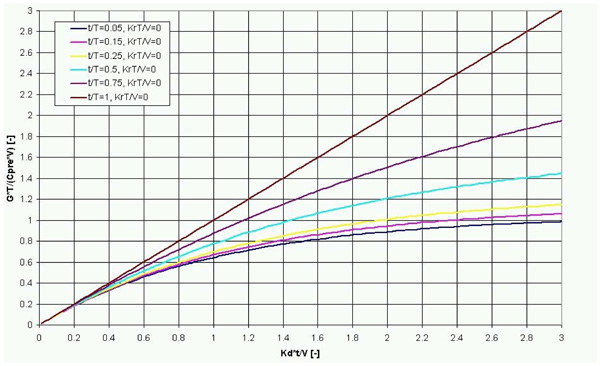Fig. (1). GT/(CpreV) (or 1/Π3a) versus Kdt/V (or Π1) with constant t/T (or Π2) and KrT/V=0. The benefit of increasing Kt/V a given amount is small for high values of Kt/V if t/T is small (t/T<0.5). This figure is similar to the “Gotch Kt/V-standardized Kt/V plot” (compare with Fig. 10), as GT/(CpreV) is linearly related to Standardized Kt/V for a constant T (see Equation 29). It should be noted that t/T=1 yields the trivial solution (CK/G=1), which represents continous dialysis.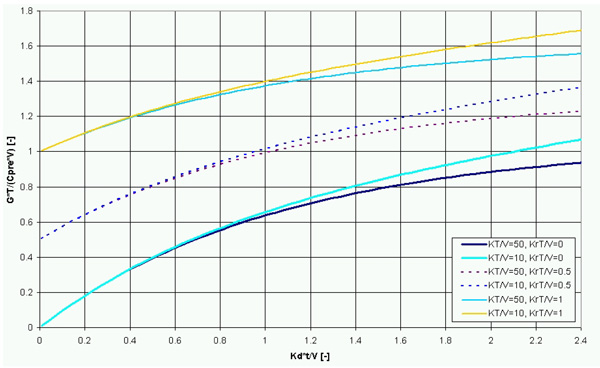Fig. (2). GT/( CpreV) (or 1/Π3a) versus Kdt/V (or Π1) with constant KdT/V (or Π1a) and KrT/V (Π4a) curves. The benefit of increasing Kt/V a given amount is small for high values of Kt/V. KrT/V and Kt/V values are approximately additive for Kt/V<1.8, as GT/(CpreV) is only weakly dependent on KT/V.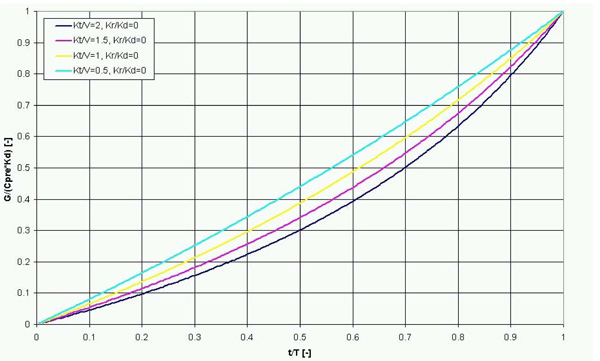Fig. (3). G/(CpreK) (or 1/Π3) versus t/T (or Π2) with constant Kdt/V (or Π1). Kr/Kd=0. This figure corresponds with Equation 18.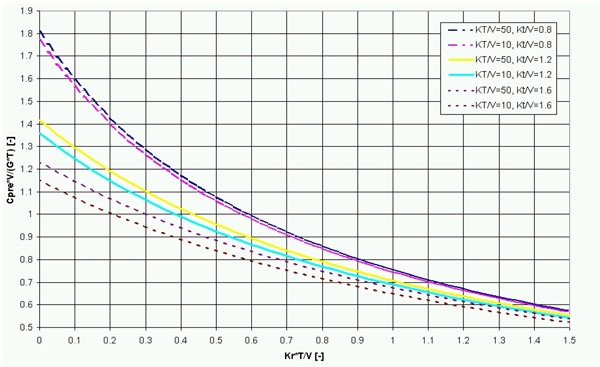Fig. (4). CpreV/(GT) versus KrT/V with constant KdT/V and Kdt/V. If the dialysis treatment parameters (t, T, K) and (other) physiologic parameters (G and V) remain the same (in conventional dialysis), as Kr decreases from a typical initial value of 10 ml/min, the Cpre increases approximately two fold.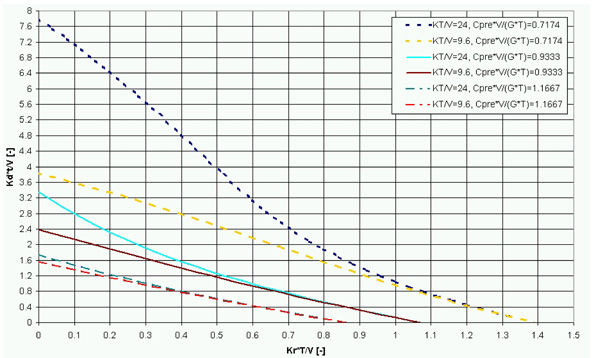Fig. (5). Kdt/V (or Π1) versus KrT/V (or Π4a) with constant KdT/V (or Π1a) and CpreV/(GT) (or Π3a). This plot demonstrates how Kdt/V would have to change to maintain the same pre-dialysis concentration if Kr declines and the other parameters (KdT/V, CpreV/(GT)) remain constant.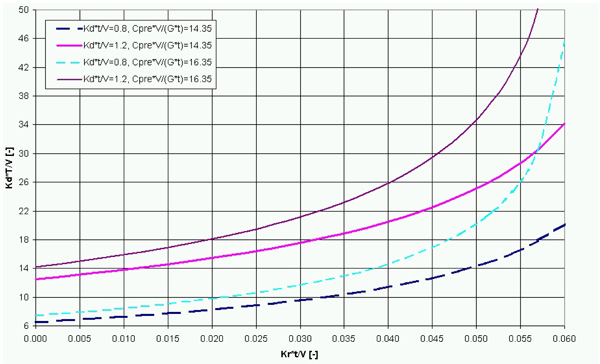Fig. (6). KdT/V (or Π1a) versus KrT/V (or Π4a) with constant CpreV/(Gt) (or Π3a/Π2) and Kdt/V (or Π1). This plot demonstrates how KdT/V would have to change to maintain the same pre-dialysis concentration if Kr declines and the other parameters (Kdt/V, CpreV/(Gt) remain constant.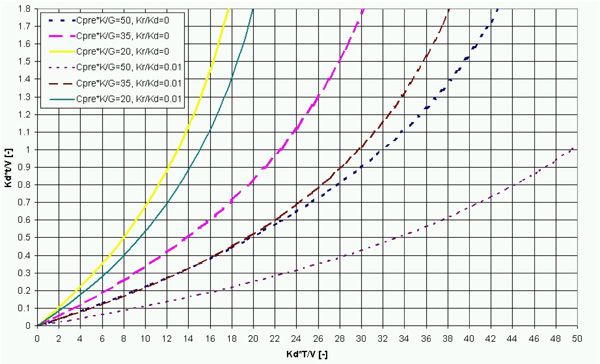Fig. (7). KdT/V (or Π1a) versus Kdt/V (or Π1) with constant CpreKd/G and Kr/Kd curves. This plot can be interpreted as the sets of t and T for constant Cpre, if other parameters are constant.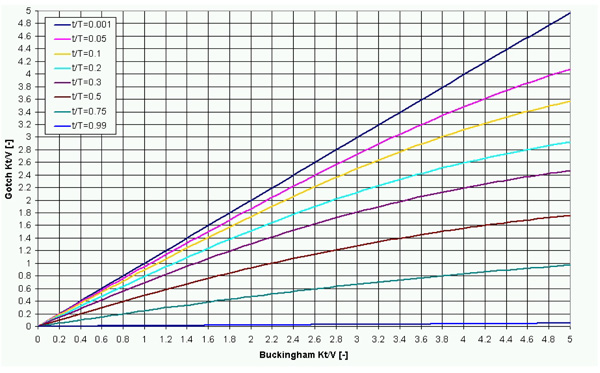Fig. (8). Gotch's Kt/V versus the Buckingham Kt/V with constant t/T, when RRF=0. If (Buckingham’s) Kt/V (or Π1) is small it is approximately equal to Gotch's Kt/V, for small values of t/T (or Π2). Gotch's Kt/V is dependent on T and deviates more from K(d)t/V with larger values of t/T and an increasing value of K(d)t/V. This figure is a plot of Equation 25.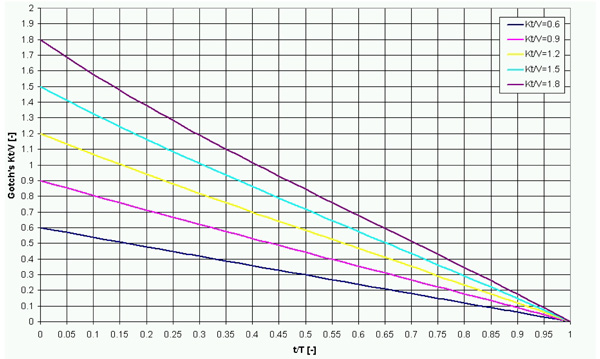Fig. (9). Gotch's Kt/V versus t/T (or Π2) with constant K(d)t/V (or Π1). Gotch's Kt/V goes zero as t/T approaches unity. Kr=0. This figure is a plot of Equation 25.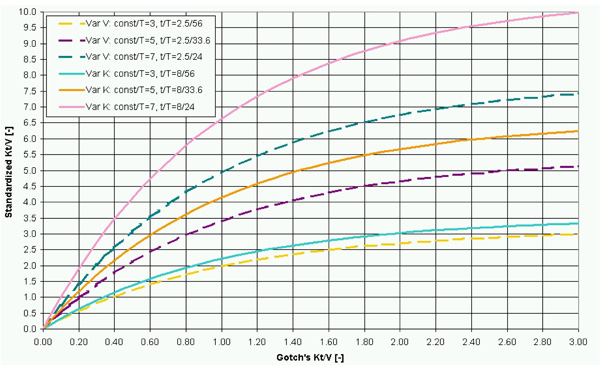Fig. (10). Standardized Kt/V versus Gotch's Kt/V. There are two sets of curves with fixed const/T values. In the first set (Var V) t=2.5 h, K=259.289 ml/min and V is varied. In the second set (Var K) t=8 h, V=40.6 L and K is varied. The spread between the two sets of lines increases as t/T increases (compare with Fig. 1) and as Kt/V increases. It should be noted that the Var V and Var K curves are only dependent on const/T and t/T; in other respects they are the same.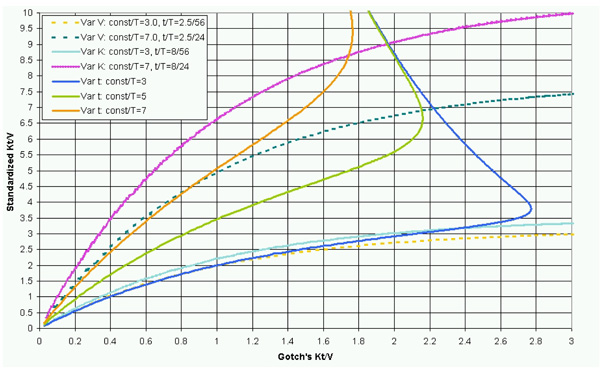Fig. (11). Standardized Kt/V versus Gotch's Kt/V. There are three sets of curves with constant T values. In the first set (Var V) t=2.5 h, K=259.289 ml/min and V is varied. In the second set (Var K) t=8 h, V=40.6 L and K is varied. In the last set (Var t) V=40.6 L, K=259.289 ml/min and t is varied. One should take note of the fact that the lines of the third set (Var t) cross the lines of the other two sets and represent curves of constant KT/V. All of the Var t curves go to 64.3752 as t/T goes to unity (data not shown), as per Equation . The spread between the three sets of lines increases as t/T increases (compare with Fig. 1) and as Kt/V increases.

In Fig. (2), an alternate Pi group, KT/V (Π1a), is used in place of t/T (Π2). GT/(CpreV) (1/Π3a) is nearly independent of both t/T (Π2) and KT/V (Π1a) for small values of Kt/V (Π1). Small Kr values (e.g., 5 ml/min) have a significant impact on the GT/(CpreV) (1/Π3,pre) value, demonstrating how residual renal function influences toxin concentrations in patients. Also, it is apparent that GT/(CpreV) values do not have a unique Kdt/V and KrT/V for a given KT/V, i.e., a given GT/(CpreV) value can be obtained from different combinations of Kdt/V and KrT/V values. KdT/V values were chosen so that a range of parameters are represented, e.g., KT/V=10 (from K = 200 ml/min, T = 33.6 h and V = 40 L) and KT/V=50 (from K = 300 ml/min, T = 56 h and V = 25 L).

It also shows that the inverse of CpreV/(GT) for Kr=0 and KrT/V sum to the inverse of CpreV/(GT) for an arbitrary Kr, when KrT/V and Kt/V are small:

Equation 23: Residual function summation approximation.

${{\left(\frac{{C}_{\mathrm{pre}}V}{\mathrm{GT}}\right)}^{1}|}_{\mathrm{Kr}}{{\left(\frac{{C}_{\mathrm{pre}}V}{\mathrm{GT}}\right)}^{1}|}_{\mathrm{Kr}=0}+\left(\frac{{K}_{r}T}{V}\right)$

if Kdt/V ≤ 1.5 and KrT/V ≤ 1.0

The data in Figs. (1-2) can also be plotted as t/T (Π2) versus G/(CpreK) (1/Π3,pre) as shown in Fig. (3). This formhas the advantage that both the ordinate and abscissa, in a physiologic context (for the case of zero RRF), vary only from zero to unity. The relation describing the lines is given by Equation 18. As (CpreK)/G (or Π3,pre) represents the maximal concentration gradient, it is apparent that temporal gradients are higher if Kt/V (Π1) is high and when t/T (Π2) is low. The maximal concentration gradient has implications in the context of dialysis cycle time and treatment time.

For non-zero residual renal function, it is useful to plot G/(CK) (1/Π3) versus t/T (Π2) with constant Kt/V (Π1) and Kr/Kd4) curves. However, it should be noted that the curves for larger values of Kr/Kd4) do not 'collapse' on the RRF=0 curves, i.e., the difference is not constant for small values of Kt/V (data not shown), like in Fig. (2).

Fig. (4) shows the impact of KrT/V on CpreV/(GT), for various values of KT/V and Kt/V. These results demonstrate the importance of residual renal function: following the run in period, the predialysis concentration is approximately two fold higher in complete renal failure (Kr = 0), compared to the case where KrT/V ~ 1.0 (when dialysis is first initiated). If Kt/V values are smaller, a proportionally larger increase in pre-dialysis concentration is observed with loss of RRF (compare Kt/V=0.8 versus Kt/V=1.2).

Fig. (5) shows the Kdt/V required to maintain a constant CpreV/(GT) as KrT/V declines. If Kd, T, V, Cpre and G are invariant, Fig. (5) represents how t (the dialysis time) must be adjusted when Kr (residual clearance) declines. As the contribution of residual renal function (Kr) declines, much more aggressive dialysis is needed if the same pre-dialysis concentration is to be maintained.

As residual renal function declines, the dialysis cycle time would (ideally) be adjusted in order to maintain the same pre-dialysis toxin concentrations. Fig. (6) thus shows the KT/V value required to maintain a constant CpreV/(Gt) as Krt/V declines. If Kd, t, V, Cpre and G are constant, Fig. (6) can be used to predict how T (cycle time) would need to be adjusted when Kr declines.

Alternatively, a consistent pre-dialysis concentration can be maintained by adjusting both the dialysis time and the cycle time. Fig. (7) thus shows the set of t and T values that lead to a constant pre-dialysis concentration, when other parameters are constant.

The conventional dialysis parameter, Gotch's Kt/V, is related to Kt/V (NOS), as shown in Fig. (8) with constant t/T curves. When t/T (Π2) is small, Kt/V (Π1) is approximately equal to Gotch's Kt/V. However, Gotch's Kt/V is only appropriate as a comparative measure of dialysis adequacy for a fixed cycle time. As shown in Fig. (8), owing to its dependence on T (or t/T (Π2)), Gotch's Kt/V increasingly deviates from Kt/V (Π1) as t/T (Π2) increases, and thus fails to adequately represent the peak pre-dialysis toxin concentration if the cycle time is adjusted.

Fig. (9) is similar to Fig. (8); it shows how Gotch's Kt/V varies with t/T (Π2) and constant (Buckingham) Kt/V (Π1) curves. Given that Gotch's Kt/V reflects the concentration fluctuation, Gotch’s Kt/V goes to zero as t/T (Π2) approaches unity. Indirectly, it demonstrates that Gotch’s Kt/V decreases with increasing frequency of dialysis. If the total dialysis time within a week is constant and frequency (1/T) is increased, one would expect that this would lead to lower toxin concentrations and better outcomes (plot in supplemental materials).

A figure similar to Fig. (9) can be created for the normalized fluctuation of concentration (NFC) that, likewise, shows that the NFC decreases with increasing t/T, independent of the Kt/V (Π1) value (plot in supplemental materials).

## Discussion

The alternate non-dimensional groups (Π1a, Π3a) are important as they allow the equations to be re-cast to better understand the relationships between the different variables. For example, the exponential relationship between dialysis time (t) and pre-dialysis concentration (Cpre) is seen in Fig. (2), and is also obvious if Fig. (2) is re-plotted with the inverse of the ordinate (CpreV/(GT)) (plot shown in supplemental material). However, the same non-dimensional groups cannot be used to understand the relationship between dialysis time (t) and the cycle time (T) because Π4a (KrT/V) contains T (as shown in Fig. (7)).

If the residual renal function is zero, three non-dimensional groups are sufficient to define a patient's dialysis intervention (as defined by their physiologic parameters (V, C, G) and their treatment parameters (t, T, K)). Thus, Figs. (1 to 3) represent different ways of plotting the same physical system (Equation 22, Equation 39) and are a family of equations related by variable transformations (such as Equation 10 and Equation 11).

The meaning and significance of the non-dimensional groups are described in detail below and are summarized in Table 2.

### Understanding Kt/V (Π1)

Physically, Kt/V (=(Kr+Kd)t/V or Kdt/V if Kr<<Kd) can be interpreted as a ratio of the treatment time and (intradialytic) system time constant; V/K is equal to the time constant describing the intradialytic transient behaviour of the system.

On a physiologic basis, Kt/V (Π1) can be interpreted as being proportional to the ratio between the dialysis time and the time, t1/2, required to complete half of the change to reach the steady state concentration, i.e., the solution to Equation 3 for t=∞ and Kr=0 is C(∞)=G/Kd (also known as the ‘wearable kidney’ concentration):

Equation 24: Kt/V interpretation.

$\frac{\mathrm{Kt}}{V}=\mathrm{ln}\left(2\right)\frac{t}{{t}_{12}}$

Where:

t =  dialysis time

t1/2 =  the time required for the concentration to be reduced halfway to C from Cpre, i.e. (Cpre+C)/2, defined as patient-dialysis machine system half-life.

C =  G/ Kd.

As a half-life (t1/2) can be recast as a time constant, Kt/V can also be interpreted as a ratio of the dialysis time and a time constant (see Appendix 3A).

The practical implication is:

• If Kt/V is high, the change in concentration (toward the steady-state value) in the latter stages of dialysis will be very small (Fig. 2).
• f t/τ is small, the change in concentration (toward the steady-state value) throughout the dialysis will be relatively large.

There are three corollaries to this:

1. More frequent dialysis treatment for short time periods results in more optimal dialysis, i.e., short daily hemodialysis is more efficient as it makes use of the steeper part of the concentration decay curve. This was found experimentally , and discussed by Depner .
2. Short daily dialysis (6x/week x 2h) and conventional hemodialysis (3x/week x 4h) have the same t/T value, but have different Kt/V values. A lower Kt/V can be better, i.e. be associated with lower toxin concentrations.
This can be seen from Fig. (3), as G/(CK) is larger for smaller values of t, if t/T, G, K and V are the same. This could also be demonstrated by plotting Equation 19 (not shown), and is illustrated with an example:
If V=35 L, K=235 ml/min (14.1 L/h), G=X [mol/h], and one compares the schedule 1 (t=1.5 h, T=28 h (6x/week)), with schedule 2 (t=3 h, T=56 h (3x/week)) then:
Schedule 1:
Kt/V= 14.1 L/h * 1.5 h / 35 L = 0.60 [-]
t/T= 1.5 h / 28 h = 0.054 [-]
CpreV/(GT) = 2.2 [-] (by Equation 19 or Fig. (2)
Cpre = 2.2 / 35 L * X mol/h * 28 h = 1.7X mol/L
Schedule 2:
Kt/V = 14.1 L/h * 3 h / 35 L = 1.2 [-]
t/T= 3 h / 56 h = 0.054 [-]
CpreV/(GT) = 1.4 [-] (by Equation 19 or Fig. (2)
Cpre = 1.4 / 35 L * X mol/h * 56 h = 2.2X mol/L
Schedule 1 (6x per week) is characterized by lower toxin concentrations. Clinically, Williams et al.  found that these patients did better.
It should be noted that the above calculation can be represented by a non-dimensional plot of CpreKd/G versus KdT/V, with curves of constant tfii/Tfi, where Tfi is a fixed time interval (e.g. a week) and tfii, is the total dialysis time (e.g. 9 hours) in the fixed time interval Tfi (data in supplemental materials).
3. Matching the concentration fluctuation and mean concentration for different V values (with constant K) requires adjustment of both t and T. This follows from the fact that a scaling of the time axis does not change the concentration fluctuation or mean concentration.

### Kt/V (Π1) and Gotch's Kt/V

Gotch's Kt/V represents two things:

1. a way to approximately scale for large and small volumes of distribution, and
2. a normalization for the urea mass generation, to remove its variability.

Physically, Gotch’s Kt/V represents the pre-dialysis to post-dialysis delta in concentration. It is dependent on the cycle time, which may appear to be counterintuitive, as 'T' does not appear in 'Kt/V'.

Kt/V (Π1) cannot directly be expressed as the concentration fluctuation, without further information (see Equation 12). Concentration fluctuation is not a function of Kt/V and only indirectly dependent on K if there is no residual renal function. Kt/V (Π1) is not dependent on T.

A relation between the two groups is (using Equation 16-Equation 19):

Equation 25: Relation between Gotch's Kt/V and Buckingham Kt/V (Π1)

Equation 25 demonstrates that Gotch’s Kt/V depends upon the cycle time, which is part of Π2.

Based on the Buckingham Pi Theorem, it is evident that Gotch’s Kt/V derives its predictive power from its relation to the non-dimensional group Π1, to which it is approximately proportional in conventional hemodialysis schedules (see Fig. 8). As such, we believe Gotch’s Kt/V should be considered an approximation of the dimensionless Kt/V (Π1), and has some utility for estimating the intradialytic time constant (described in Appendix 3A) when t/T (Π2) is small.

A comparison between Gotch's Kt/V and Kt/V (Π1) is presented in Table 3.

### Understanding C/(GT/V) (Π3a)

GT is the mass generation during the interdialytic cycle time T. If GT is divided by V, it becomes a concentration. This concentration is equivalent to the change in concentration during the cycle time (T) if K=0. Therefore, CV/(GT) represents the concentration divided by the anephric rise in the concentration during the cycle time T. Alternatively, CV/(GT) can also be considered to be the ratio of (toxin) mass in the body to (toxin) mass generated in the time ‘T’.

### Understanding CK/G (Π3)

Physically, CK/G represents a ratio between the mass removal and mass generation. CK/G=1 (Π3=1) represents the outcome for a wearable kidney, where C is the steady state concentration, K the clearance and G the mass generation rate (see Equation 18, Fig. (3). Most nephrologists are familiar with the Cockcroft-Gault equation; it represents the case where CK/G=1, or K=G/C, where K is the patient’s clearance.

For conditions where t/T (Π2) does not equal unity, CK/G (Π3) is equal to a multiple of the wearable kidney concentration.

If one re-arranges G/(CK) and recognizes that K can be interpreted as a rate constant (k) divided by the control volume (V), it can be cast as ratio of rate constants (see Appendix 3B).

If Cpre is chosen as the reference concentration and K is the dialyzer clearance, G/(CK) represents the mean mass generation relative to the peak mass removal rate in the cycle (if RRF=0). Thus, G/ CpreK reflects the maximal (temporal concentration) gradients encountered during dialysis; small values (<<1) represent high temporal gradients and values near unity represent minimal temporal gradients.

### Understanding t/T (Π2)

If the RRF is zero, t/T (P2) is equal to unity less the ratio of the actual concentration change during the cycle divided by the maximum rise in concentration during the cycle. In other words, it is the concentration fluctuation avoided by dialysis divided by the maximum concentration fluctuation during a given cycle time T (from Equation 13):

Equation 26: t/T in terms of concentration fluctuation avoided, if Kr/Kd=0.

Where:

Cpost-Cpre is the toxin concentration change due to dialysis.

GT/V is the anephric rise in toxin concentration (the maximum fluctuation for a given T).

### Understanding Kr/Kd (Π4)

Kr/Kd characterizes the impact of RRF in hemodialysis. It relates the mass turnover for a wearable kidney to the mass turnover due to the native kidney.

### Understanding KrT/V (Π4A)

KrT/V can be understood as a ratio of the cycle time and interdialytic time constant. Like Kr/Kd, it characterizes the impact of RRF, and represents the fractional removal of a substance from the control volume during the cycle time.

### Gotch’s Kt/V and Standardized Kt/V

Gotch has published a figure, which relates Gotch’s Kt/V and standardized Kt/V (see Gotch et al. [13, 19]). We believe this is a pseudo non-dimensional form of Fig. (1).

Standardized Kt/V, which represents a normalized concentration (C/(G/V)), is dependent on t and K separately. When t approaches T (i.e., Π2→1), Gotch’s Kt/V goes to zero (see Fig. 9). As shown in Fig. (1), when K becomes large, or V becomes small, the concentration (Cpre) decreases by a very small amount; the slope (GT/(CpreV))/(Kt/V), or (1/Π3a)/Π1, is small for large values of Kt/V (i.e., Π1>2) and values (Π2)<0.25. Physiologically, this occurs because the dialysis is so effective that it drives the toxin concentration to near zero, and the behavior of the system is therefore governed predominantly by the concentration rise in the interdialytic period.

Gotch et al. [13, 19] present the concept of continuous clearance, and incorrectly illustrate this concept by failing to recognize that their results are based upon an implicitly assumed cycle time (T) and dialysis time (t). Continuous clearance, i.e., t/T=1, is in fact characterized by a Gotch Kt/V equal to zero (see Equation 1). Using Equation 2 and Equation , one can show that:

Equation 27: Standardized Kt/V, if t/T=1.

The Gotch figures were created by varying K or V, but the lines that Gotch et al. claim to represent weekly frequency really represent constant lines of T and t/T, which have some similarity to Fig. (1). Using Equation 19 and Equation 25, it is possible to show that:

Equation 28: Relation between Π3a, Π2 and Gotch's Kt/V for Kr=0.

${}_{3a}=\frac{1{}_{2}}{1{e}^{}}$

Where:

Ψ is Gotch’s Kt/V

Since CpreV/GT can be transformed, with some multiplication, to standardized Kt/V, it can be shown (using Equation 2, Equation 19 and Equation 25) that:

Equation 29: Standardized (Std) Kt/V and Gotch's Kt/V for Kr=0.

Fig. (11) shows the relationship between standardized Kt/V and Gotch’s Kt/V, while independently varying K, t and V (for several constant values of T). Fig. (10) shows how standardized Kt/V depends on const/T, Gotch’s Kt/V, and t/T, similar to a figure developed by Leypold et al. .

The variation of K and V yields the same set of curves; the variables K and 1/V are interchangeable (see Fig. (10). However, the variables K and t (or 1/V and t) cannot be treated interchangeably, as t is also present in Π2. Also, it should be noted that the ‘T var’ curves cannot be written as a mathematical function of Gotch’s Kt/V, as most values of Gotch’s Kt/V represent two Standardized Kt/V values, i.e., the ‘T var’ curves fail the vertical line test.

The Gotch figure thus does not completely capture the true relationship between the quantities plotted (Gotch’s Kt/V and standardized Kt/V) and may be misleading. Consequently, the relationship between Gotch’s Kt/V and standardized Kt/V may be poorly understood among engineers and nephrologists.

### Normalized Fluctuation of Concentration (NFC) and URR

Physically, NFC (defined by Equation 15) can be interpreted as the anephric concentration rise between dialysis sessions relative to the pre-dialysis concentration (in the dynamic equilibrium state), if the dialysis sessions are equally spaced and there is no residual renal function.

Urea reduction ratio (URR) is equal to the NFC, if the concentrations considered are those of urea.

As the anephric rise is dependent on the length of the interdialytic time, decreased spacing of dialysis sessions with the same Kt/V for a given patient, i.e., constant G and V, will lead to a decrease in URR, because the anephric rise in concentration between dialysis treatments has been reduced (see Fig. 9) and the supplemental materials.

### Peritoneal Dialysis, Non-Dimensional Groups and Kt/V (Π1)

Lysaght developed an expression for Kt/V for peritoneal dialysis . However, Lysaght’s Kt/V is not related to the dimensionless Kt/V (Π1), but rather, is more closely related to Gotch's standardized Kt/V and Gotch's Kt/V for hemodialysis (see Equation 2).

Owing to their distinctly different definitions, it is not appropriate to compare Gotch's Kt/V and Lysaght's Kt/V, which, unfortunately, makes it difficult for nephrologists to compare patient outcomes from peritoneal dialysis and hemodialysis.

### Standardized Kt/V and Lysaght's Kt/V for Peritoneal Dialysis

The definitions of Standardized Kt/V and Lysaght’s Kt/V are similar (compare Equation 2 and Equation 9), but neither are bona fide non-dimensional groups. Standardized Kt/V represents a concentration expressed relative to G/V, with a time factor (604,800 seconds) to make it look like a genuine non-dimensional group (see Equation 2). Lysaght’s Kt/V is similar but lacks the adjustment factor; Lysaght’s definition of Kt/V for peritoneal dialysis actually has units of 1/time .

### Sufficiency

In the case of zero residual renal function, there are three non-dimensional groups. The Buckingham Pi Theorem therefore suggests that there should be a function fn2 that relates the three Pi groups:

Equation 30: Relation of Pi groups.

If two Pi groups are known, the third can be determined using the function fn2 or a function related to fn2. We have illustrated this using several equations that relate Π1, Π2, and various forms of Π3 or Π3a.

Kt/V (Π1) by itself is insufficient to determine Π3a, as demonstrated via Equation 11, which shows that Π3a depends upon Π1, Π2, and Π3, and by Equation 19, which shows that Π3a depends upon Π1, and Π2. If the cycle time, T, is assumed or fixed, as is frequently done, knowledge of Π1 (K, t and V) is sufficient to solve for C/(GT/V) (Π3a).

Equation 19 is a good first approximation of fn2 when C is Cpre; it also demonstrates that there only is a weak dependence on t/T (Π2) for the range of parameters typical in conventional hemodialysis (K=200-300 ml/min, t=2-4 hours, T=56 hours).

### Approximately Constant G/V

Using the non-dimensional analysis and the mass transfer model, it is possible to surmise that G/V is approximately constant in a group of individuals for each of the most important toxins associated with end-stage renal disease (ESRD), if RRF=0:

If Kt/V is fixed (e.g., 1.2) and T is fixed (e.g., 56 h), CpreV/(GT) will be essentially invariant (for typical Vs and Ks), as it only weakly depends on t/T, as shown via Fig. (1) and Equation 19.

If the pre-dialysis concentration (Cpre) of uremic toxins is predictive of survival, it should be similar for people with a similar survival. Thus, in conventional dialysis, Cpre is invariant for a given Kt/V and T. However, if T is altered, or if K changes due to changes in RRF, Cpre can increase or decrease. Nonetheless, for a given Kt/V and T, once T, Cpre, and CpreV/GT are fixed, it follows that G/V must be invariant. This can be shown algebraically; using Equation 3 and Equation 4 (for the case of Kr=0), it is possible to show that Gotch’s Kt/V (Equation 1) and Standardized Kt/V (Equation 2) are independent of G/V.

Direct measurement of toxin concentration is required to verify this. It is possible that a subset of patients have a G/V that deviates significantly from the norm; such a “high G/V subset” would have high toxin concentrations, despite being “dialyzed well” by matching Gotch’s Kt/V.

### Scaling for Size

Kt/V (like Gotch’s Kt/V) does not scale perfectly for patient size, unless t/T is also matched. If one assumes K, G/V and T are constants, it is apparent from a plot of Equation 19 that the concentration is not perfectly matched as t/T changes. Increasing t/T leads to a decreased CV/(GT), which must result in a lower C, if Kt/V, G and T are constant and V is increased.

Thus, smaller individuals on (conventional) hemodialysis theoretically have slightly higher toxin concentrations, if treatment is based on matching Kt/V or Gotch’s Kt/V (instead of matching toxin concentrations) and K values are equal.

If one assumes that Kt/V=1.20, K=250 ml/min, and T=56 h, and two individuals are compared with a V of 40 L and 25 L, the t values will be 3.2 h and 2 h respectively. The values of CV/(GT) (or Π3a) for the two individuals will be 1.397 and 1.410 respectively, based on Equation 19.

This difference is likely not clinically significant. Also, larger individuals typically can tolerate slightly higher blood flow rates and thus have slightly higher K values than smaller individuals, meaning that the difference is often smaller that suggested by the above calculation.

### Residual Renal Function

Residual renal function is known to be a predictor of survival. Fig. (4) shows that the concentration of toxins increases approximately two fold from initial prescription of renal dialysis to complete kidney failure.

Figs. (5 and 6) show that the intensity of dialysis required to compensate for the loss of RRF is significant. If an individual with V=35 L and initial renal clearance Kr = 8 mL/min is conventionally dialyzed thrice weekly (i.e., T=56 h, with a Kd=250 ml/min to a target of Kt/V=0.9 at dialysis initiation), once complete renal failure occurs (Kr = 0), the required Kt/V to maintain the same (toxin) concentration would be more than five times higher, at 4.71 (by iterative solution of Equation 22 – calculation in supplemental material). If the same person were dialyzed 5x weekly, the Kt/V would have to be approximately 1.14. Alternatively, the same pre-dialysis toxin concentration could be achieved by reducing T to 29.1 h, while keeping Kt/V constant at 0.9.

Toxin concentrations in individuals on dialysis without RRF are significantly higher than those with some RRF, unless the cycle time and dialyzer clearance are adjusted to compensate. One could speculate that it is not coincidence that the loss of RRF is correlated with the reduction in median survival.

It has been speculated that the HEMO study failed as it did not have a sufficient separation between the high dose and low dose groups , and we believe that this, in part, was a result of noise due to RRF. The RRF exclusion criterion in the study was >1.5 ml/min/(35 L). If this exclusion criterion is multiplied by the cycle time (56 h), one obtains a KrT/V value of 0.144. For the two Kt/V values that were compared (1.32 versus 1.73), it is apparent from Fig. (4) that similar values for CpreV/(GT) can be obtained for the RRF range in the study: CpreV/(GT) = 1.19 for Kt/V=1.32, KT/V=28.8 and KrT/V=0.144, versus CpreV/(GT) = 1.18 for Kt/V=1.73, KT/V=28.8 and KrT/V=0.

The NCDS RRF exclusion criterion was even higher (3 ml/min) and it can be shown using Fig. (4) that the toxin concentrations in the high intensity (long dialysis time) group and low intensity (short dialysis time) group likely overlapped.

The role of residual function was not well appreciated by nephrologists at the time of NCDS and the HEMO study. We believe this is due to the fact that it is non-trivial to calculate, and its contribution not apparent if dialysis adequacy is assessed using Gotch’s Kt/V. This issue is resolved if (1) measures of dialysis are based toxin concentrations and (2) the dimensionless mass transfer analysis developed in this paper is employed as a guide to design dialysis schedules and understand the effect of residual renal function on toxin concentration.

### Dialysis Measurement

The pseudo nondimensional groups (Gotch’s Kt/V, Lysaght’s Kt/V, Standardized Kt/V) were important steps forward approximately twenty-five years ago, and a significant improvement over what preceded them (measuring urea concentration); yet, they are impractical measures of dialysis. They are (1) nontrivial to calculate, (2) their interpretation, as indicators of (toxin) concentration, is complicated, and (3) it is not possible to directly compare patients with normal renal function and pre-dialysis patients to patients receiving dialysis treatment. Indeed, one cannot even compare different dialysis modalities (peritoneal dialysis, hemodiafiltration, hemodialysis), making it difficult to recommend the most appropriate/effective treatment regimen.

We believe that dialysis measurement should progress into a post-urea era. It should be based on toxin concentrations, and should be independent of treatment modality, in the same way that control of blood sugar in a diabetic is focused on achieving a target blood sugar level, even though there are several possible ways to achieve that goal (e.g., glyburide, metformin, lifestyle modification, insulin).

### Limitations

The mass transfer model used in this manuscript assumes that there is no volume change. Volume change during dialysis is a factor known to affect the hemodynamics, but, generally, does not appreciably affect the concentrations. Post-dialysis concentration rebound was not addressed.

We are aware that Gotch’s equation for Kt/V has been superceded by equations developed by Daugirdas. We believe that Daugirdas’ equation is, essentially, Equation 1 with corrections for effects from (1) post-dialysis concentration rebound and (2) interdialytic volume change.

While toxin shifts between body compartments may be relevant in the pathophysiology of ESRD, the goal of the rebound calculation in urea mass transfer modeling is to find the pre-dialysis to post-dialysis delta, so that K/V can be estimated. Also, it is not proven that the gradients following dialysis are physiologically more important than the gradients in the intradialytic period.

Both volume change during the cycle and multiple pool models can be analyzed with a non-dimensional approach; however, they are beyond the scope of this work.

We have assumed that there is equal spacing of dialysis, as is commonly done [13, 16]. This is not true, but accounting for the actual treatment intervals (e.g., M/W/F) complicates the analysis significantly and we found in the past that it does not significantly alter the conclusions (data not shown).

## CONCLUSIONS

### Insights from Non-Dimensional Analysis

This paper has demonstrated the value of non-dimensional groups in dialysis, and has illustrated the physical interpretations of these groups.

Non-dimensional forms of the mass transfer equations can confirm a number of well known experimental findings, which may not be immediately apparent on examination of the governing equations (Equation 3 and Equation 4):

1. Increasing dialysis frequency leads to lower toxin concentrations for a fixed total dialysis time within a week.
2. RRF has a considerable effect on pre-dialysis concentration (see Fig. 4); thus, it is not surprising that RRF is a predictor of survival.
3. The toxin concentration, expressed as the toxin mass generation rate (G) divided by the volume of distribution (V), is approximately constant in a group of individuals for the most important toxins individually associated with end-stage renal disease.

Plots were created using the dimensionless groups that characterize hemodialysis, to facilitate understanding of the dominant factors/parameters in hemodialysis, including:

1. The change in dialysis dose required to replace lost RRF is considerable – a factor of five or more (Figs. 5 and 6).
2. There is a set of schedules based on the dialysis dose and time between treatments that can achieve the same pre-dialysis toxin concentration (Fig. 7).
3. Gotch’s Kt/V and URR decrease with increased t/T values (see Fig. 9 and supplemental materials), which makes their interpretation more difficult as the dialysis frequency is varied. A lower URR may result in lower toxin concentrations when the frequency of dialysis is higher.

### Implications of the Findings

We believe the assumption of constant G/V implicit in Gotch’s Kt/V should be investigated through direct measurement of toxins, as a subset of patients may have an abnormal G/V and thus are disadvantaged by matching Gotch’s Kt/V.

Our model analysis also provides a possible reason for the null outcome for the HEMO and NCDS studies; it is possibly due to effects of residual renal function leading to similar pre-dialysis toxin concentrations.

The adoption of Gotch’s Kt/V may have led to a focus on dialysis time per session instead of toxin concentration, dialysis frequency, fluid balance and patient well being. We believe it is time to re-focus on (toxin) concentrations. The EUTox group  has developed a large list of compounds that are elevated in renal failure. These concentrations in renal failure are presented as a ratio relative to the concentrations found in healthy individuals. It is time for physicians to re-double their efforts to identify the compounds responsible for the mortality and morbidity of renal disease, so that engineers can build better dialyzers to target the important toxins and biochemists and physicians can develop targeted pharmaceuticals. The dimensionless mass transfer model presented can aid in this effort, as it makes it easier to follow toxin concentrations and show how they vary under different treatment modalities. The dimensionless groups enable scaling for patient size and a greater understanding of complex interactions; however, non-dimensional groups do not replace dimensional values.

### Bottom Line Conclusion

Dialysis modalities (e.g. peritoneal dialysis, hemodialysis) should be quantified the same way, as shown via the theoretical development presented in this paper. This requires a transition from the historical measures independently developed for each treatment modality. In fact, we believe that the different measures to assess dialysis adequacy have hindered direct comparisons between hemodialysis and peritoneal dialysis, and have also hindered understanding of end-stage renal disease.

It is time to move towards objective, quantitative measures of dialysis adequacy based on the concentrations of toxins, or concentrations of toxins normalized by the concentrations of those toxins found in healthy individuals.

## AUTHORS’ CONTRIBUTIONS

MB conceived the study, did the calculations, created the figures and wrote the first draft of the manuscript.

BAS contributed to the development of the concepts central to the analysis, critically revised the manuscript and reviewed the calculations.

## ACKNOWLEDGEMENTS

MB would like to thank the nephrology staff at St. Michael’s Hospital in Toronto and Joanne M. Bargman from the University Health Network for teaching him about clinical aspects of nephrology and inspiring this work.

## Appendix

### APPENDIX 1A

If the limit Kr→0 is applied to Equation 3 and Equation 4:

Equation 31: Dialytic equation – functional form.

$C\left({t}_{\mathrm{var}}\right)=\frac{G}{K}+{\left({C}_{\mathrm{pre}}\frac{G}{K}\right)e}^{\frac{{\mathrm{Kt}}_{\mathrm{var}}}{V}}$

Equation 32: Interdialytic equation – functional form.

$C\left({t}_{\mathrm{var}}\right)=\frac{G}{V}{t}_{\mathrm{var}}+{C}_{\mathrm{post}}$

### APPENDIX 1B

An analytic form to determine the pre- and post-dialysis concentrations can be derived by applying the boundary conditions to the functional form (Equation 31 and Equation 32):

Equation 33: Dialytic equation without RRF - analytic form.

${C}_{\mathrm{post}}=\frac{G}{K}+\left({C}_{\mathrm{pre}}\frac{G}{K}\right){e}^{\frac{\mathrm{Kt}}{V}}$

Equation 34: Interdialytic equation without RRF - analytic form.

${C}_{\mathrm{pre}}=\frac{G}{V}\left(Tt\right)+{C}_{\mathrm{post}}$

Where t is the duration of dialysis, and T is the dialysis cycle time. Other variables are defined in the text, and/or are described in Table 1.

If Equation 33 is substituted into Equation 34 the result is:

Equation 35: Interdialytic equation without RRF and without Cpost.

${C}_{\mathrm{pre}}=\frac{G}{V}\left(Tt\right)+\frac{G}{K}+\left({C}_{\mathrm{pre}}\frac{G}{K}\right){e}^{\frac{\mathrm{Kt}}{V}}$

If the above is multiplied by K/G, the result is:

Equation 36: Non-dimensional form of interdialytic equation without RRF and without Cpost..

$\frac{{C}_{\mathrm{pre}}K}{G}=\frac{\mathrm{Kt}}{V}\frac{T}{t}\frac{\mathrm{Kt}}{V}+1+\left(\frac{{C}_{\mathrm{pre}}K}{G}1\right){e}^{\frac{\mathrm{Kt}}{V}}$

Using Equation 5, Equation 6, Equation 7, the above equation becomes:

Equation 37: Alternate form of Equation 18.

${}_{3}=\frac{{}_{1}}{{}_{2}}{}_{1}+1+\left({}_{3}1\right){e}^{{}_{1}}$

It is possible to show that Equation 37 is Equation 18, as the following is true:

${e}^{{}_{1}}=\frac{1}{{e}^{{}_{1}}}$

### APPENDIX 1C

Equation 12 in a form without Kt/V:

Equation 38: Dimensionless form 2 of Equation 3 (Kr=0).

### APPENDIX 2

Equation 39: Alternate form of relation of non-dimensional groups with residual renal function - compare with Equation 22.

${}_{3a}=\frac{\frac{1}{{}_{4a}}+\left(\frac{1}{{}_{4a}+{}_{2a}}\left(1{A}_{k}\right)\frac{1}{{}_{4a}}\right){B}_{k}}{1{A}_{k}{B}_{k}}$

Where:

$\begin{array}{c}{A}_{k}=exp\left({}_{1}\left(\frac{{}_{4a}}{{}_{2a}}1\right)\right),{B}_{k}=\mathrm{exp}\left({}_{4a}\left(\frac{{}_{1}}{{}_{2a}}1\right)\right)\\ {}_{1}=\frac{{K}_{d}t}{V},{}_{2a}=\frac{{K}_{d}T}{V},{}_{3a}=\frac{{C}_{\mathrm{pre}}V}{\mathrm{GT}},{}_{4a}=\frac{{K}_{r}T}{V}\end{array}$

### APPENDIX 3A

Equation 40: Alternate Kt/V interpretation.

$\frac{\mathrm{Kt}}{V}=\frac{t}{}$

Where:

τ =   the intradialytic time constant of the patient-hemodialysis machine system and the time to complete 63.2% of the change to the wearable kidney concentration, C(∞).

t =   dialysis time.

### APPENDIX 3B

If one re-arranges G/(CK) and recognizes that K can be interpreted as a rate constant (k) divided by the control volume (V), it can be cast as ratio of rate constants:

Equation 41: G/(CK) as ratio of rate constants.

$\frac{\left(\frac{G}{\mathrm{CV}}\right)}{k}$

Where:

G/(CV) =  mass generation per mass (in the control volume) or the fractional mass generation rate.

k =  fraction of mass removed per time

It can be noted that in a biological context, such as bacterial growth, G/(CV) may be constant. In dialysis, this is not believed to be the case, as the waste products in the body (organic and inorganic compounds) are themselves not thought to produce additional waste.

### SUPPLEMENTAL MATERIAL

Supplementary material is available on the publisher's Web site along with the published article.

Calculations_24.xls -contains some additional figures.

Loss_of_RFF_calc6.xls - contains loss of RRF calculation.

## REFERENCES

  Shinaberger J H. “Quantitation of dialysis: historical perspective,” Semin Dial 2001; 14: 238-45.  Babb A L, Popovich R P, Christopher T G, and Scribner B H. “The genesis of the square meter-hour hypothesis,” Trans Am Soc Artif Intern Organs 1971; 17: 81-91.  Sargent J A. “Control of dialysis by a single-pool urea model: the national cooperative dialysis study,” Kidney Int Suppl 1983; 13: S19-25.  Burgelman M, Vanholder R, Fostier H, and Ringoir S. “Estimation of parameters in a two-pool urea kinetic model for hemodialysis,” Med Eng Phys 1997; 19: 69-76.  White F M, Ed. Fluid mechanics. 3rd. Toronto: McGraw-Hill Inc 1994.  Buckingham E. “On physically similar systems; illustrations of the use of dimensional equations,” Phys Rev 1914; 4: 345-76.  Oertel H Jr. Stroemungsmechanik: methoden und phaenomene 1995.  Buckingham E. “The principle of similitude,” Nature 1915; 96: 396-7.  Buckingham E. “Model experiments and the forms of empirical equations,” Trans ASME 1915; 37: 263-96.  Gotch F A, and Sargent J A. “A mechanistic analysis of the national cooperative dialysis study (ncds),” Kidney Int 1985; 28: 526-34.  National Kidney Foundation. “Clinical practice guidelines for hemodialysis adequacy, update 2006,” Am J Kidney Dis 2006; 48(Suppl 1): S2-90.  Eknoyan G, Beck G J, Cheung A K, et al. “Effect of dialysis dose and membrane flux in maintenance hemodialysis,” N Engl J Med 2002; 347: 2010-9.  Gotch F A. “The current place of urea kinetic modelling with respect to different dialysis modalities,” Nephrol Dial Transp 1998; 13(Suppl 6): 10-4.  Roberson J A, Crowe C T. Enginering fluid mechanics. 6th. New York: John Wiley & Sons Inc 1997.  James M L, Smith G M, Wolford J C. Applied numerical methods for digital computation 4th. 1993.  Depner T A. “Quantifying hemodialysis and peritoneal dialysis: examination of peak concentration hypothesis,” Semin Dial 1994; 7: 315-7.  Williams A W, Chebrolu S B, Ing T S, et al. “Early clinical, quality-of-life, and biochemical changes of "daily hemodialysis" (6 dialyses per week),” Am J Kidney Dis 2004; 43: 90-102.  Depner T A. “Benefits of more frequent dialysis: lower tac at the same Kt/V,” Nephrol Dial Transplant 1998; 13(Suppl. 6): 20-4.  Gotch F A, Sargent J A, Keen M L. “Whither goest kt/v?” Kidney Int Suppl 2000; 76: S3-18.  Leypoldt J K, Jaber B L, and Zimmerman D L. “Predicting treatment dose for novel therapies using urea standard kt/v,” Semin Dial 2004; 17: 142-5.  Lysaght M J, Pollock C A, Hallet M D, Ibels L S, Farrell P C. “The relevance of urea kinetic modeling to CAPD,” ASAIO Trans 1989; 35: 784-90.  Scribner B H, and Blagg C R. “Effect of dialysis dose and membrane flux in maintenance hemodialysis,” N Engl J Med 2003; 348: 1491-4. author reply 1491-4  Vanholder R, Smet R De, Glorieux G, et al. “Review on uremic toxins: classification, concentration, and interindividual variability,” Kidney Int 2003; 63: 1934-43.